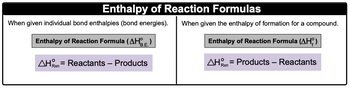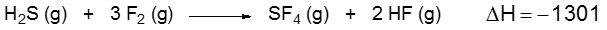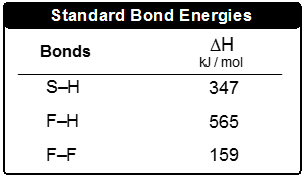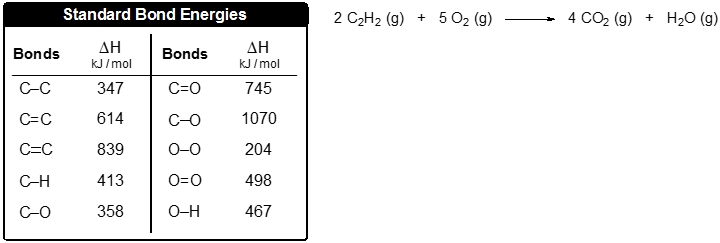Start typing, then use the up and down aroows to select an option from the list.

# GOB Chemistry

Learn the toughest concepts covered in your GOB - General, Organic, and Biological Chemistry class with step-by-step video tutorials and practice problems.

Energy, Rate and Equilibrium

Bond Energy is the amount of energy stored within a chemical bond.

## Bond Energy

1

### concept

Bond Energy1m
Play a video:
0

When individual bond energies are given, we will use the first formula.2

### example

Bond Energy Example 12m
Play a video:
0
3
Problem

Consider the following equation:Determine the bond enthalpy value for the F–S bond.4
Problem

Use the bond energies to estimate the enthalpy of reaction for the combustion of 5 moles of acetylene: